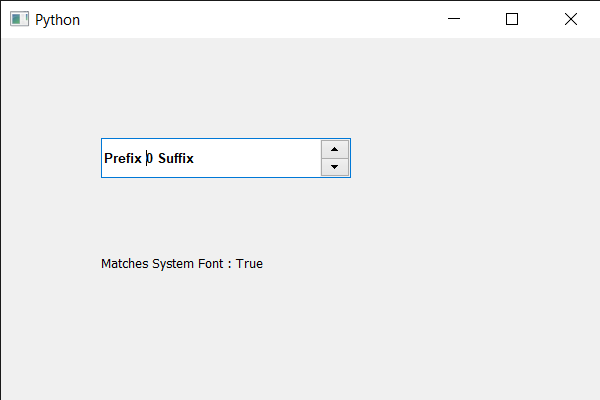Related Articles
PyQt5 QSpinBox – Matching font with the System Font
• Last Updated : 22 May, 2020

In this article we will see how we can match the font of the spin box with the system font, Matching of font means it is similar to that of the system font. We can get the QFont object of spin box with the help of `font` method.

Note : Make text italic or bold doesn’t mean the font has changed, by default system has Arial font. If we set different font from Arial then it will return False output as they don’t match.

In order to do this we use exactMatch method with the QFont object of the spin box

Syntax : font.exactMatch()

Argument : It takes no argument

Return : It returns bool

Below is the implementation

 `# importing libraries``from` `PyQt5.QtWidgets ``import` `*` `from` `PyQt5 ``import` `QtCore, QtGui``from` `PyQt5.QtGui ``import` `*` `from` `PyQt5.QtCore ``import` `*` `import` `sys`` ` ` ` `class` `Window(QMainWindow):`` ` `    ``def` `__init__(``self``):``        ``super``().__init__()`` ` `        ``# setting title``        ``self``.setWindowTitle(``"Python "``)`` ` `        ``# setting geometry``        ``self``.setGeometry(``100``, ``100``, ``600``, ``400``)`` ` `        ``# calling method``        ``self``.UiComponents()`` ` `        ``# showing all the widgets``        ``self``.show()`` ` `        ``# method for widgets``    ``def` `UiComponents(``self``):``        ``# creating spin box``        ``self``.spin ``=` `QSpinBox(``self``)`` ` `        ``# setting geometry to spin box``        ``self``.spin.setGeometry(``100``, ``100``, ``250``, ``40``)`` ` `        ``# setting range to the spin box``        ``self``.spin.setRange(``0``, ``999999``)`` ` `        ``# setting prefix to spin``        ``self``.spin.setPrefix(``"Prefix "``)`` ` `        ``# setting suffix to spin``        ``self``.spin.setSuffix(``" Suffix"``)`` ` `        ``# getting font of the spin box``        ``font ``=` `QFont(``'Arial'``)`` ` `        ``# making font bold``        ``font.setBold(``True``)`` ` `        ``# setting this font to the spin box``        ``self``.spin.setFont(font)`` ` `        ``# checking if font matches with the system font``        ``match ``=` `font.exactMatch()`` ` `        ``# creating a label``        ``label ``=` `QLabel(``self``)`` ` `        ``# setting geometry to the label``        ``label.setGeometry(``100``, ``200``, ``300``, ``50``)`` ` `        ``# setting text to the label``        ``label.setText(``"Matches System Font : "` `+` `str``(match))`` ` ` ` `# create pyqt5 app``App ``=` `QApplication(sys.argv)`` ` `# create the instance of our Window``window ``=` `Window()`` ` `# start the app``sys.exit(App.``exec``())`

Output :Attention geek! Strengthen your foundations with the Python Programming Foundation Course and learn the basics.

To begin with, your interview preparations Enhance your Data Structures concepts with the Python DS Course. And to begin with your Machine Learning Journey, join the Machine Learning – Basic Level Course

My Personal Notes arrow_drop_up